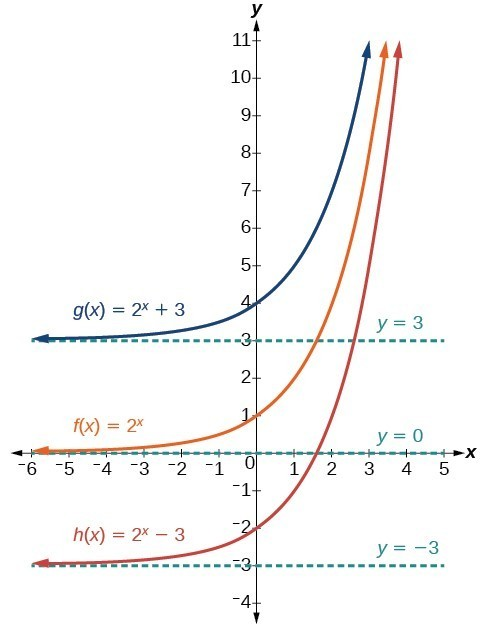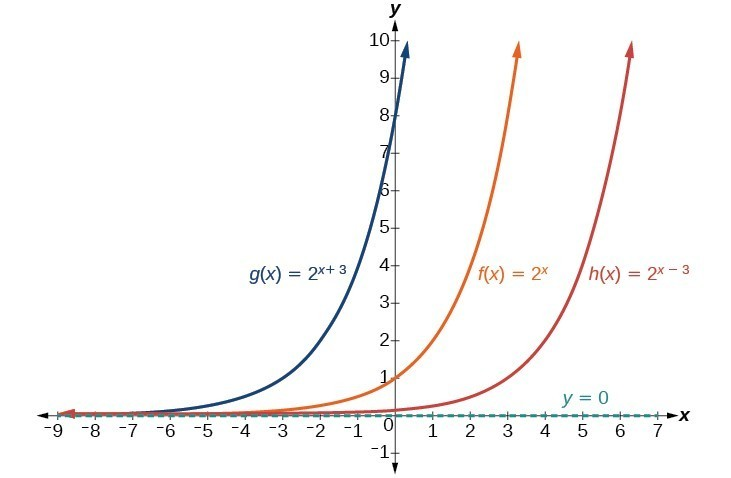## Horizontal and Vertical Translations of Exponential Functions

### Learning Outcomes

• Graph exponential functions shifted horizontally or vertically and write the associated equation.

Transformations of exponential graphs behave similarly to those of other functions. Just as with other parent functions, we can apply the four types of transformations—shifts, reflections, stretches, and compressions—to the parent function $f\left(x\right)={b}^{x}$ without loss of shape. For instance, just as the quadratic function maintains its parabolic shape when shifted, reflected, stretched, or compressed, the exponential function also maintains its general shape regardless of the transformations applied.

## Graphing a Vertical Shift

The first transformation occurs when we add a constant d to the parent function $f\left(x\right)={b}^{x}$ giving us a vertical shift d units in the same direction as the sign. For example, if we begin by graphing a parent function, $f\left(x\right)={2}^{x}$, we can then graph two vertical shifts alongside it using $d=3$: the upward shift, $g\left(x\right)={2}^{x}+3$ and the downward shift, $h\left(x\right)={2}^{x}-3$. Both vertical shifts are shown in the figure below.Observe the results of shifting $f\left(x\right)={2}^{x}$ vertically:

• The domain $\left(-\infty ,\infty \right)$ remains unchanged.
• When the function is shifted up 3 units giving $g\left(x\right)={2}^{x}+3$:
• The y-intercept shifts up 3 units to $\left(0,4\right)$.
• The asymptote shifts up 3 units to $y=3$.
• The range becomes $\left(3,\infty \right)$.
• When the function is shifted down 3 units giving $h\left(x\right)={2}^{x}-3$:
• The y-intercept shifts down 3 units to $\left(0,-2\right)$.
• The asymptote also shifts down 3 units to $y=-3$.
• The range becomes $\left(-3,\infty \right)$.

## Graphing a Horizontal Shift

The next transformation occurs when we add a constant c to the input of the parent function $f\left(x\right)={b}^{x}$ giving us a horizontal shift c units in the opposite direction of the sign. For example, if we begin by graphing the parent function $f\left(x\right)={2}^{x}$, we can then graph two horizontal shifts alongside it using $c=3$: the shift left, $g\left(x\right)={2}^{x+3}$, and the shift right, $h\left(x\right)={2}^{x - 3}$. Both horizontal shifts are shown in the graph below.Observe the results of shifting $f\left(x\right)={2}^{x}$ horizontally:

• The domain, $\left(-\infty ,\infty \right)$, remains unchanged.
• The asymptote, $y=0$, remains unchanged.
• The y-intercept shifts such that:
• When the function is shifted left 3 units to $g\left(x\right)={2}^{x+3}$, the y-intercept becomes $\left(0,8\right)$. This is because ${2}^{x+3}=\left({2}^{3}\right){2}^{x}=\left(8\right){2}^{x}$, so the initial value of the function is 8.
• When the function is shifted right 3 units to $h\left(x\right)={2}^{x - 3}$, the y-intercept becomes $\left(0,\frac{1}{8}\right)$. Again, see that ${2}^{x-3}=\left({2}^{-3}\right){2}^{x}=\left(\frac{1}{8}\right){2}^{x}$, so the initial value of the function is $\frac{1}{8}$.

### A General Note: Shifts of the Parent Function $f\left(x\right)={b}^{x}$

For any constants c and d, the function $f\left(x\right)={b}^{x+c}+d$ shifts the parent function $f\left(x\right)={b}^{x}$

• shifts the parent function $f\left(x\right)={b}^{x}$ vertically d units, in the same direction as the sign of d.
• shifts the parent function $f\left(x\right)={b}^{x}$ horizontally c units, in the opposite direction as the sign of c.
• has a y-intercept of $\left(0,{b}^{c}+d\right)$.
• has a horizontal asymptote of yd.
• has a range of $\left(d,\infty \right)$.
• has a domain of $\left(-\infty ,\infty \right)$ which remains unchanged.

### How To: Given an exponential function with the form $f\left(x\right)={b}^{x+c}+d$, graph the translation

1. Draw the horizontal asymptote yd.
2. Shift the graph of $f\left(x\right)={b}^{x}$ left c units if c is positive and right $c$ units if c is negative.
3. Shift the graph of $f\left(x\right)={b}^{x}$ up d units if d is positive and down d units if d is negative.
4. State the domain, $\left(-\infty ,\infty \right)$, the range, $\left(d,\infty \right)$, and the horizontal asymptote $y=d$.

### Example: Graphing a Shift of an Exponential Function

Graph $f\left(x\right)={2}^{x+1}-3$. State the domain, range, and asymptote.

### Try It

Sketch a graph of the function $f\left(x\right)={2}^{x - 1}+3$. State domain, range, and asymptote.

In the following video, we show more examples of the difference between horizontal and vertical shifts of exponential functions and the resulting graphs and equations.

## Using a Graph to Approximate a Solution to an Exponential Equation

Graphing can help you confirm or find the solution to an exponential equation. For example,$42=1.2{\left(5\right)}^{x}+2.8$ can be solved to find the specific value for x that makes it a true statement. Graphing $y=4$ along with $y=2^{x}$ in the same window, the point(s) of intersection if any represent the solutions of the equation.

To use a calculator to solve this, press [Y=] and enter $1.2(5)x+2.8$ next to Y1=. Then enter 42 next to Y2=. For a window, use the values –3 to 3 for$x$ and –5 to 55 for$y$.Press [GRAPH]. The graphs should intersect somewhere near$x=2$.

For a better approximation, press [2ND] then [CALC]. Select [5: intersect] and press [ENTER] three times. The x-coordinate of the point of intersection is displayed as 2.1661943. (Your answer may be different if you use a different window or use a different value for Guess?) To the nearest thousandth,x≈2.166.

### Try It

Solve $4=7.85{\left(1.15\right)}^{x}-2.27$ graphically. Round to the nearest thousandth.

## Contribute!

Did you have an idea for improving this content? We’d love your input.